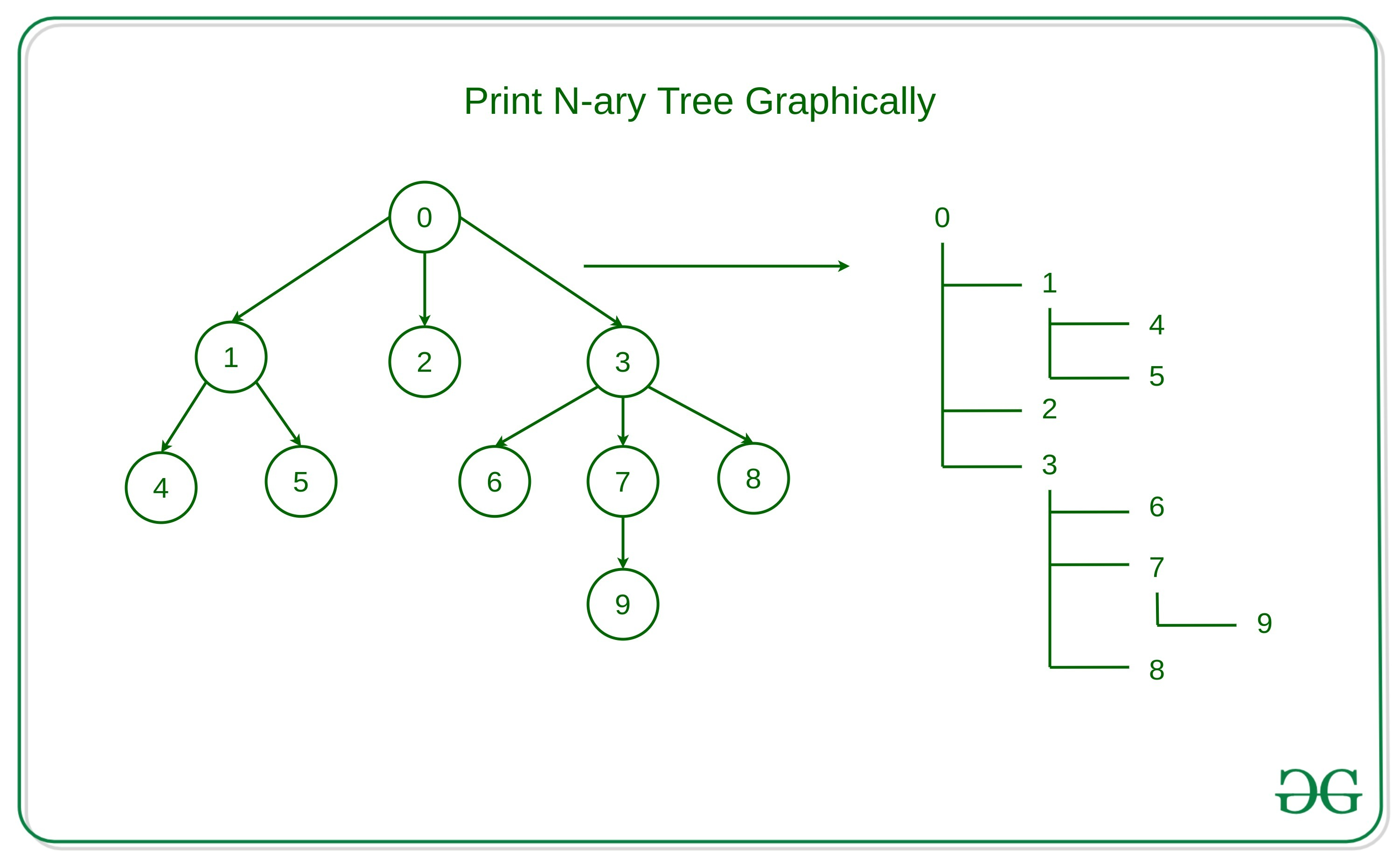# Print N-ary tree graphically

Given an N-ary tree, the task is to print the N-ary tree graphically.

Graphical Representation of Tree: A representation of tree in which the root is printed in a line and the children nodes are printed in subsequenct lines with some amount of indentation.
Examples:

```Input:
0
/ | \
/  |  \
1   2   3
/ \    / | \
4   5  6  7  8
|
9
Output:
0
+--- 1
|    +--- 4
|    +--- 5
+--- 2
+--- 3
+--- 6
+--- 7
|    +--- 9
+--- 8
```## Recommended: Please try your approach on {IDE} first, before moving on to the solution.

Approach: The idea is to traverse the N-ary Tree using DFS Traversal to traverse the nodes and explore its children nodes until all the nodes are visited and then similarly, traverse the sibling nodes.

The step-by-step algorithm for the above approach is described below –

• Intialize a variable to store the current depth of the node, for root node the depth is 0.
• Declare a boolean array to store the current exploring depths and initially mark all of them to False.
• If the current node is a root node that is the depth of the node is 0, then simply print the data of the node.
• Otherwise, Iterate over a loop from 1 to the current depth of node and print, ‘|’ and three spaces for each of the exploring depth and for non-exploring depth print three spaces only.
• Print the current value of the node and move the output pointer to the next line.
• If the current node is the last node of that depth then mark that depth as non-exploring.
• Similarly, explore all the child nodes with the recursive call.

Below is the implementation of the above approcah:

## C++

 `// C++ implementation to print ` `// N-ary Tree graphically ` ` `  `#include ` `#include ` `#include ` ` `  `using` `namespace` `std; ` ` `  `// Structure of the node ` `struct` `tnode {  ` `    ``int` `n; ` `    ``list root; ` `    ``tnode(``int` `data) ` `        ``: n(data) ` `    ``{ ` `    ``} ` `}; ` ` `  `// Function to print the ` `// N-ary tree graphically ` `void` `printNTree(tnode* x, ` `    ``vector<``bool``> flag,  ` `    ``int` `depth = 0, ``bool` `isLast = ``false``) ` `{ ` `    ``// Condition when node is None ` `    ``if` `(x == NULL)  ` `        ``return``; ` `     `  `    ``// Loop to print the depths of the ` `    ``// current node ` `    ``for` `(``int` `i = 1; i < depth; ++i) { ` `         `  `        ``// Condition when the depth  ` `        ``// is exploring ` `        ``if` `(flag[i] == ``true``) { ` `            ``cout << ``"| "` `                ``<< ``" "` `                ``<< ``" "` `                ``<< ``" "``; ` `        ``} ` `         `  `        ``// Otherwise print  ` `        ``// the blank spaces ` `        ``else` `{ ` `            ``cout << ``" "` `                ``<< ``" "` `                ``<< ``" "` `                ``<< ``" "``; ` `        ``} ` `    ``} ` `     `  `    ``// Condition when the current ` `    ``// node is the root node ` `    ``if` `(depth == 0) ` `        ``cout << x->n << ``'\n'``; ` `     `  `    ``// Condtion when the node is  ` `    ``// the last node of  ` `    ``// the exploring depth ` `    ``else` `if` `(isLast) { ` `        ``cout << ``"+--- "` `<< x->n << ``'\n'``; ` `         `  `        ``// No more childrens turn it  ` `        ``// to the non-exploring depth ` `        ``flag[depth] = ``false``; ` `    ``} ` `    ``else` `{ ` `        ``cout << ``"+--- "` `<< x->n << ``'\n'``; ` `    ``} ` ` `  `    ``int` `it = 0; ` `    ``for` `(``auto` `i = x->root.begin();  ` `    ``i != x->root.end(); ++i, ++it) ` ` `  `        ``// Recursive call for the ` `        ``// children nodes ` `        ``printNTree(*i, flag, depth + 1,  ` `            ``it == (x->root.size()) - 1); ` `    ``flag[depth] = ``true``; ` `} ` ` `  `// Function to form the Tree and  ` `// print it graphically ` `void` `formAndPrintTree(){ ` `    ``int` `nv = 10; ` `    ``tnode r(0), n1(1), n2(2),  ` `        ``n3(3), n4(4), n5(5), ` `    ``n6(6), n7(7), n8(8), n9(9); ` `     `  `    ``// Array to keep track  ` `    ``// of exploring depths ` `    ``vector<``bool``> flag(nv, ``true``); ` `     `  `    ``// Tree Formation ` `    ``r.root.push_back(&n1); ` `    ``n1.root.push_back(&n4); ` `    ``n1.root.push_back(&n5); ` `    ``r.root.push_back(&n2); ` `    ``r.root.push_back(&n3); ` `    ``n3.root.push_back(&n6); ` `    ``n3.root.push_back(&n7); ` `    ``n7.root.push_back(&n9); ` `    ``n3.root.push_back(&n8); ` ` `  `    ``printNTree(&r, flag); ` `} ` ` `  `// Driver Code ` `int` `main(``int` `argc, ``char` `const``* argv[]) ` `{ ` `     `  `    ``// Function Call ` `    ``formAndPrintTree(); ` `    ``return` `0; ` `} `

Output:

```0
+--- 1
|    +--- 4
|    +--- 5
+--- 2
+--- 3
+--- 6
+--- 7
|    +--- 9
+--- 8
```

Performance Analysis:

• Time Complexity: In the above-given approach, there is recusive call to explore all the vertices which takes O(V) time. Therefore, the time complexity for this approach will be O(V).
• Auxiliary Space Complexity: In the above-given approach, there is extra space used to store the exploring depths. Therefore, the auxiliary space complexity for the above approach will be O(V)

Attention reader! Don’t stop learning now. Get hold of all the important DSA concepts with the DSA Self Paced Course at a student-friendly price and become industry ready.

My Personal Notes arrow_drop_upCheck out this Author's contributed articles.

If you like GeeksforGeeks and would like to contribute, you can also write an article using contribute.geeksforgeeks.org or mail your article to contribute@geeksforgeeks.org. See your article appearing on the GeeksforGeeks main page and help other Geeks.

Please Improve this article if you find anything incorrect by clicking on the "Improve Article" button below.

Improved By : nidhi_biet